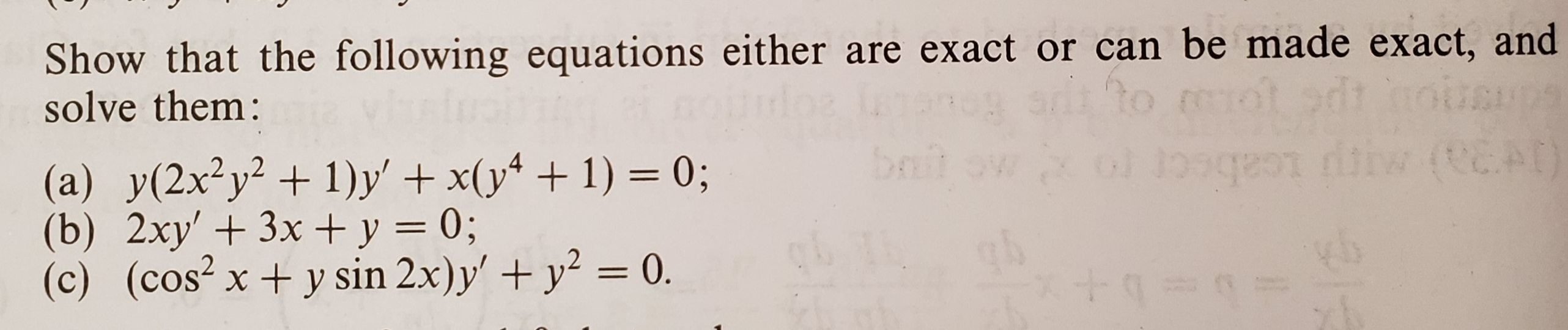# Show that the following equations either are exact or can be made exact, andsolve them:2,,2(b) 2xy' 3x y 0;(c) (cos2x +y sin 2x)y'+y0sin 2x) v'

Question
118 viewshelp_outlineImage TranscriptioncloseShow that the following equations either are exact or can be made exact, and solve them: 2,,2 (b) 2xy' 3x y 0; (c) (cos2x +y sin 2x)y'+y0 sin 2x) v' fullscreen
check_circle

Step 1

To modify (if necessary) the given equations into exact form and thus solve the equation

Step 2

A differential equation is called exact if it can be written in the form d(f(x,y)) =0 , in which case it can be integrated as f(x,y)=C (so y can be solved at least as an implicit function x).

Step 3

A general differential equation might not be presented in exact form; but at times, by suitable manipulation , one could bring it to an exact fo...

### Want to see the full answer?

See Solution

#### Want to see this answer and more?

Solutions are written by subject experts who are available 24/7. Questions are typically answered within 1 hour.*

See Solution
*Response times may vary by subject and question.
Tagged in

### Math